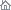Home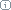About Us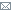ContactBlogWhat's NewProductsBuy NowDownloadsForumGeneXproTools Online Guide Learn how to use the 5 modeling platforms of GeneXproTools with the Online GuideLast update: February 19, 2014ROC Analysis

Receiver Operating Characteristic or ROC Curves are powerful visualization tools that allow a quick assessment of the quality of a model. They are usually plotted in reference to a Baseline or Random Model, with the Area Under the ROC Curve (or AUC for short) as a widely used indicator of the quality of a model.

So, for the Random Model, the area under the ROC curve is equal to 0.5, which means that the further up (or down, for inverted models) a model is from 0.5 the better it is. Indeed, for perfect models on both sides of the random line, what is called ROC heaven takes place when AUC = 1 (for normal models) or AUC = 0 (for inverted models). Below is shown a typical ROC curve obtained for a risk assessment model using a training dataset with 18,253 cases. This model, which has a classification accuracy of 74.15% and an R-square of 0.2445 (R-square values might seem unusually low, but in risk assessment applications R-square values around 0.22 are considered excellent and indicative of a good model), has an AUC of 0.7968. Note that the classification accuracy reported refers to the accuracy of the logistic regression model, not the ROC accuracy evaluated using the ROC Cutoff Point.

Below is shown a Gallery of ROC Curves typical of intermediate models generated during a GeneXproTools run. These ROC curves were specifically created for a risk assessment problem with a training dataset with 18,253 cases and using a small population of just 30 programs. The Classification Accuracy, the R-square, and the Area Under the ROC Curve (AUC) of each model, as well as the generation at which they were discovered, are also shown as illustration. From top to bottom, they are as follow (see also the twin Gallery of Logistic Fit Charts in the Logistic Fit section):

 Generation 0, Accuracy = 65.33%, R-square = 0.0001, AUC = 0.5273 Generation 5, Accuracy = 66.03%, R-square = 0.0173, AUC = 0.5834 Generation 59, Accuracy = 66.92%, R-square = 0.0421, AUC = 0.6221 Generation 75, Accuracy = 68.99%, R-square = 0.1076, AUC = 0.7068 Generation 155, Accuracy = 69.93%, R-square = 0.1477, AUC = 0.7597 Generation 489, Accuracy = 74.15%, R-square = 0.2445, AUC = 0.7968Generation 0, Accuracy = 65.33%, R-square = 0.0001, AUC = 0.5273Generation 5, Accuracy = 66.03%, R-square = 0.0173, AUC = 0.5834Generation 59, Accuracy = 66.92%, R-square = 0.0421, AUC = 0.6221Generation 75, Accuracy = 68.99%, R-square = 0.1076, AUC = 0.7068Generation 155, Accuracy = 69.93%, R-square = 0.1477, AUC = 0.7597Generation 489, Accuracy = 74.15%, R-square = 0.2445, AUC = 0.7968

ROC Curves and ROC Tables are also useful to evaluate what is called the Optimal Cutoff Point, which is given by the maximum of the Youden index. The Youden index J returns the maximum value of the expression (for inverted models, it returns the minimum):

J = max[SE(t) + SP(t) - 1]

where SE(t) and SP(t) are, respectively, the sensitivity and specificity over all possible threshold values t of the model. Thus, the ROC Cutoff Point corresponds to the model output at the Optimal Cutoff Point.

In the ROC Table, GeneXproTools also shows all “SE + SP -1” values and highlights in light green the row with the Optimal Cutoff Point and corresponding ROC Cutoff Point. These parameters are also shown in the ROC Statistics Report.

The ROC Cutoff Point can be obviously used to evaluate a Confusion Matrix (in the Logistic Regression Window it is called ROC Confusion Matrix in order to distinguish it from the Logistic Confusion Matrix) and, in the Cutoff Points Table, you have access to the Predicted Class, the Match, and Type values used to build the ROC Confusion Matrix (you can see the graphical representation of the ROC Confusion Matrix in the Confusion Matrix section).

The visualization of the ROC Confusion Matrix is a valuable tool and can be used to determine the right number of bins to achieve a good fit with the Logistic Regression Model. But GeneXproTools allows you to do more with the ROC Confusion Matrix and associated ROC Cutoff Point. By allowing the conversion of Logistic Regression runs to the Classification Framework, you can use this model, with its finely adapted ROC Cutoff Point, straightaway to make binary classifications using the Classification Scoring Engine of GeneXproTools. Note, however, that you'll have to change the Rounding Threshold to ROC Threshold in the Settings Panel (when a Logistic Regression run is converted to Classification, the Rounding Threshold is set to Logistic Threshold by default) and then recalculate all model thresholds by selecting Update All Thresholds in the History menu.

The Youden index is also used to evaluate a wide range of useful statistics at the Optimal Cutoff Point (OCP statistics for short). They include:

• TP (True Positives)
• TN (True Negatives)
• FP (False Positives)
• FN (False Negatives)
• TPR (True Positives Rate or Sensitivity)
• TNR (True Negatives Rate or Specificity)
• FPR (False Positives Rate, also known as 1-Specificity)
• FNR (False Negatives Rate)
• PPV (Positive Predictive Value)
• NPV (Negative Predictive Value)
• Classification Accuracy (Correct Classifications)
• Classification Error (Wrong Classifications)

How they are calculated is shown in the table below ("TC" represents the number of Total Cases):

 TPR (Sensitivity) TP / (TP + FN) TNR (Specificity) TN / (TN + FP) FPR (1-Specificity) FP / (FP + TN) FNR FN / (FN + TP) PPV TP / (TP + FP), and TP + FP ≠ 0 NPV TN / (TN + FN), and TN + FN ≠ 0 Classification Accuracy (TP + TN) / TC Classification Error (FP + FN) / TC

It is worth pointing out that OCP statistics are quantile-independent and are therefore a good indicator of what could be achieved with a model in terms of logistic fit and accuracy.

Related Tutorials:

Related Videos:

 Leave Feedback Please enter the number below using the combo boxes before sending your feedback. 3 8 4 0 1 2 3 4 5 6 7 8 9   0 1 2 3 4 5 6 7 8 9   0 1 2 3 4 5 6 7 8 9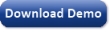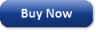Time Limited Trial

Try GeneXproTools for free for 30 days!

Released February 19, 2014

Last update: 5.0.3883New Entries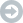Subscribe to the GEP-List

 3 8 4 0 1 2 3 4 5 6 7 8 9   0 1 2 3 4 5 6 7 8 9   0 1 2 3 4 5 6 7 8 9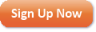Home | What's New | Products | Buy Now | Downloads | Quick Tour | Support | Contact Us | About Gepsoft | Sign UpForum | Blog | Videos | Tutorials | Server Knowledge Base | Logistic Regression Guide | Terms of Use | Privacy & Cookies